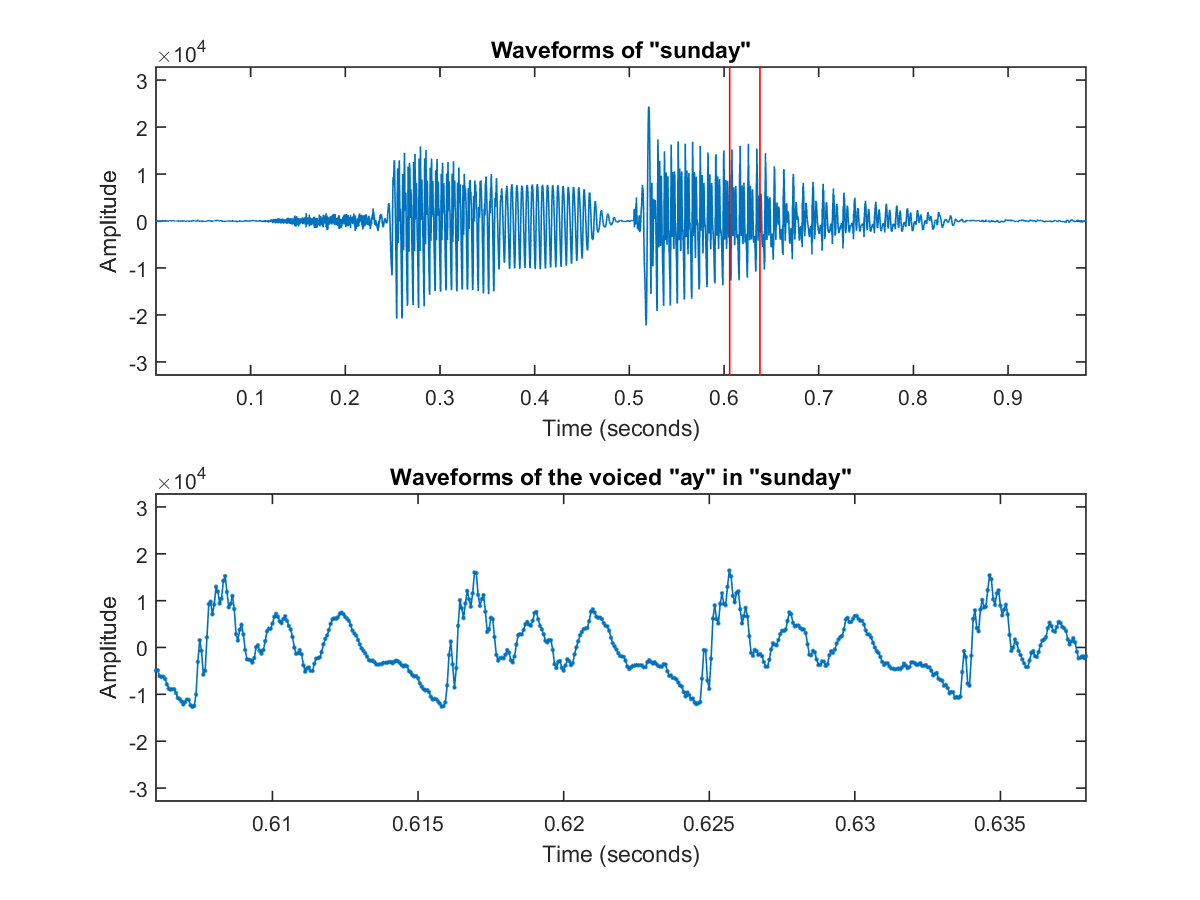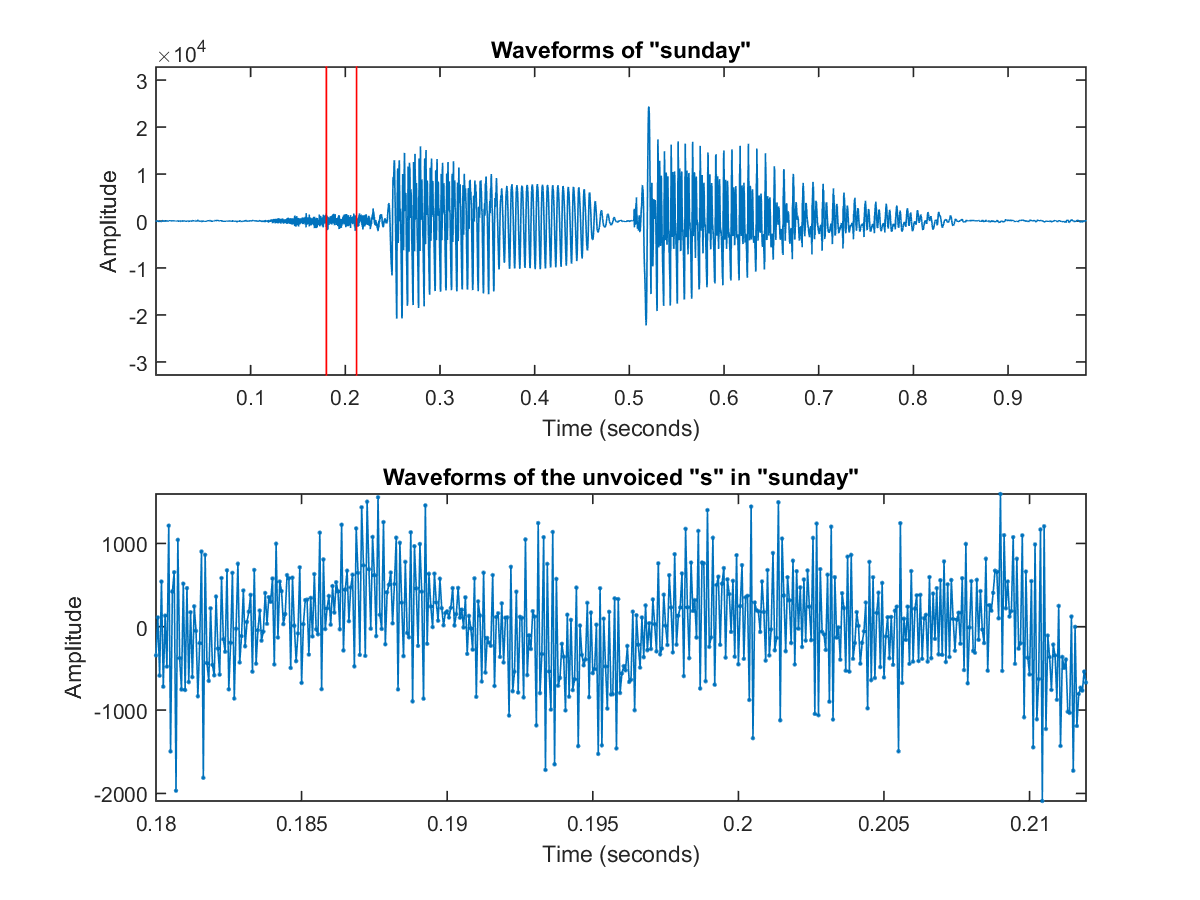## 3-1 Introduction to Audio Signals (嚙踝蕭嚙踝蕭嚙踝蕭嚙踝蕭嚙踝蕭嚙踝蕭嚙踝蕭嚙踝蕭嚙踝蕭)

[chinese][all]

Audio signals are generally referred to as signals that are audible to humans. Audio signals usually come from a sound source which vibrates in the audible frequency range. The vibrations push the air to form pressure waves that travels at about 340 meters per second. Our inner ears can receive these pressure signals and send them to our brain for further recognition.

There are numerous ways to classify audio signals. If we consider the source of audio signals, we can classify them into two categories:

• Sounds from animals: Such as human voices, dog's barking, cat's mewing, frog's croaking. (In particular, Bioacoustics is a cross-disciplinary science, which investigates sound production and reception in animals.)
• Sounds from non-animals: Sounds from car engines, thunder, door slamming, music instruments, etc.

If we consider repeated patterns within audio signals, we can classify them into another two categories:

• Quasi-periodic sound: The waveforms consist of similar repeated patterns such that we can perceive the pitch. Examples of such sounds include monophonical playback of most music instruments (such as pianos, violins, guitars, etc) and human's singing/speaking.
• Aperiodic sound: The waveforms do not consists of obvious repeated patterns so we cannot perceive a stable pitch. Examples of such sounds include thunder pounding, hand clapping, unvoiced part in a human's utterance, and so on.

In principle, we can divide each short segment (also known as frame, with a length of about 20 ms) of human's voices into two types:

• Voiced sound: These are produced by the vibration of vocal cords. Since they are produced by the regular vibration of the vocal cords, you can observe the fundamental periods in a frame. Moreover, due to the existence of the fundamental period, you can also perceive a stable pitch.
• Unvoiced sound: These are not produced by the vibration of vocal cords. Instead, they are produced by the rapid flow of air through the mouse, the nose, or the teeth. Since these sounds are produced by the noise-like rapid air flow, we can not observed the fundamenta period and no stable pitch can be perceived.
It is very easy to distinguish between these two types of sound. When you pronunce an utterance, just put your hand on your throat to see if you feel the vibration of your vocal cords. If yes, it is voiced; otherwise it is unvoiced. You can also observe the waveforms to see if you can identify the fundamental periods. If yes, it is voiced; otherwise, it is unoviced.

The following figure shows the voiced sound of "ay" in the utterance "sunday".

Example 1: voicedFrame01.mfigure; waveFile='sunday.wav'; au=myAudioRead(waveFile); y=au.signal; fs=au.fs; nbits=au.nbits; y=y*2^nbits/2; subplot(2,1,1) time=(1:length(y))/fs; plot(time, y); axis([min(time), max(time), -2^nbits/2, 2^nbits/2]); xlabel('Time (seconds)'); ylabel('Amplitude'); title('Waveforms of "sunday"'); frameSize=512; index1=0.606*fs; index2=index1+frameSize-1; line(time(index1)*[1, 1], 2^nbits/2*[-1 1], 'color', 'r'); line(time(index2)*[1, 1], 2^nbits/2*[-1 1], 'color', 'r'); subplot(2,1,2); time2=time(index1:index2); y2=y(index1:index2); plot(time2, y2, '.-'); axis([min(time2), max(time2), -2^nbits/2, 2^nbits/2]); xlabel('Time (seconds)'); ylabel('Amplitude'); title('Waveforms of the voiced "ay" in "sunday"');You can easiy identify the fundamental period in the closeup plot.

On the other hand, we can also observe the unvoiced sound of "s" in the utterance "sunday", as shown in the following example:

Example 2: unvoicedFrame01.mwaveFile='sunday.wav'; au=myAudioRead(waveFile); y=au.signal; fs=au.fs; nbits=au.nbits; y=y*2^nbits/2; subplot(2,1,1) time=(1:length(y))/fs; plot(time, y); axis([min(time), max(time), -2^nbits/2, 2^nbits/2]); xlabel('Time (seconds)'); ylabel('Amplitude'); title('Waveforms of "sunday"'); frameSize=512; index1=0.18*fs; index2=index1+frameSize-1; line(time(index1)*[1, 1], 2^nbits/2*[-1 1], 'color', 'r'); line(time(index2)*[1, 1], 2^nbits/2*[-1 1], 'color', 'r'); subplot(2,1,2); time2=time(index1:index2); y2=y(index1:index2); plot(time2, y2, '.-'); axis([min(time2), max(time2), -inf inf]); xlabel('Time (seconds)'); ylabel('Amplitude'); title('Waveforms of the unvoiced "s" in "sunday"');In contract, there is no fundamental periods and the waveform is noise-like.

Hint
You can also use CoolEdit for simple recording, replay and observation of audio signals.

Audio signals actually represent the air pressure as a function of time, which is a continuous in both time and signal amplitude. When we want to digitize the signals for storage in a computer, there are several parameter to consider.

• Sample rate: This is the number of sample points per second, in the unit of Hertz (abbreviated as Hz). A higher sample rate indicate better sound quality, but the storage space is also bigger. Commonly used sample rates are listed next:
1. 8 kHz: Voice quality for phones and toys
2. 16 KHz: Commonly used for speech recognition
3. 44.1 KHz: CD quality
• Bit resolution: The number of bits used to represent each sample point of audio signals. Commonly used bit resolutions are listed next:
1. 8-bit: The corresponding range is 0~255 or -128~127.
2. 16-bit: The corresponding range is -32768~32767.
In other words, each sample point is represented by an integer of 8 or 16 bits. However, in MATLAB, all audio signals are normalized to floating-point number within the range [-1, 1] for easy manipulation. If you want to revert to the original integer values, you need to multiply the float-point values by 2^nbits/2, where nbits is the bit resolution.
• Channels: We have mono for single channel and stereo for double channels.

Let take my utterance of sunday for example. It is a mono recording with a sample rate of 16000 (16 KHz) and a bit resolution of 16 bits (2 bytes). It also contains 15716 sample points, corresponding to a time duration of 15716/16000 = 0.98 seconds. Therefore the file size is about 15716*2 = 31432 bytes = 31.4 KB. In fact, the file size for storing audio signals is usually quite big without compression. For instance:

• If we used the same parameters for a one-minute recording, the file size will be 60 sec x 16 KHz x 2 Byte = 1920 KB, close to 2 MB.
• For audio music in a CD, we have stereo recordings with a sample rate of 44.1 KHz, a bit resolution of 16 Bits. Therefore for a 3-minute audio music, the file size is 180 sec x 44.1 KHz x 2 Byte x 2 = 31752 KB = 32 MB. (From here you will also know the MP3 compression ratio is about 10.)

Audio Signal Processing and Recognition (音訊處理與辨識)